GMAT  >  Test: Tree Structure

# Test: Tree Structure

Test Description

## 13 Questions MCQ Test Integrated Reasoning for GMAT | Test: Tree Structure

Test: Tree Structure for GMAT 2023 is part of Integrated Reasoning for GMAT preparation. The Test: Tree Structure questions and answers have been prepared according to the GMAT exam syllabus.The Test: Tree Structure MCQs are made for GMAT 2023 Exam. Find important definitions, questions, notes, meanings, examples, exercises, MCQs and online tests for Test: Tree Structure below.
Solutions of Test: Tree Structure questions in English are available as part of our Integrated Reasoning for GMAT for GMAT & Test: Tree Structure solutions in Hindi for Integrated Reasoning for GMAT course. Download more important topics, notes, lectures and mock test series for GMAT Exam by signing up for free. Attempt Test: Tree Structure | 13 questions in 40 minutes | Mock test for GMAT preparation | Free important questions MCQ to study Integrated Reasoning for GMAT for GMAT Exam | Download free PDF with solutions
 1 Crore+ students have signed up on EduRev. Have you?
Test: Tree Structure - Question 1

### 70 % of all the staff members in a college are administrators and remaining are teachers. If the total number of teachers is 60, then what is the total number of staff members in the college?

Test: Tree Structure - Question 2

### A total of 500 students applied to a college for admission to its MBA program. The applicants were either freshers or experienced professionals. If 70% of all applicants were freshers and 20% of the experienced professionals had an experience of at least 2 years, then how many experienced professionals had an experience of less than 2 years?

Detailed Solution for Test: Tree Structure - Question 2

Given Fresher + Work ex = 500
Also 70% of 500 = Fresher
Then 30% of 500 = work ex= 150

Now 20% of 150 have atleast 2 year or more work ex

So 80% of 150 will have less than 2 year work ex= 80/100 x 150 = 120

Test: Tree Structure - Question 3

### Candidate A, B and C are contesting elections. Each voter can only vote for a single candidate. 20% of the voters refrained from voting and candidates A, B and C received votes in the ratio 4 :3: 1 respectively. If there were no invalid votes and Candidate C got 2 million votes, then how many voters (in millions) refrained from voting?

Test: Tree Structure - Question 4

A book publishing company released 100 books last year. Three-fifth of the released books were fiction and all the remaining were non-fiction. 20% of fiction has at least 350 pages. And 80% of the non-fiction has less than 350 pages. How many of the released books has less than 350 pages?

Test: Tree Structure - Question 5

In a village 40% of the men are above 25-years of age. Among these, 50% are married and 60% of these married men have at least one kid. If there are a total of 72000 men who are at most 25-year-old, how many of the married men does not have any kids?

Test: Tree Structure - Question 6

A real estate broker booked 1200 apartments for sale. All of them were either 2-bedroom or 3-bedroom apartments.  Out of the 2-bedroom apartments, 20% had terrace gardens and out of the 3-bedroom apartments, 80% had terrace gardens. If there were 600 apartments with terrace gardens, how many 3-bedroom apartments were booked by the broker?

Detailed Solution for Test: Tree Structure - Question 6

Step 1: Question statement and Inferences

We are given that a broker booked 1200 apartments for sale. There are only two types of apartments in these 1200: either 2-bedroom apartments or 3-bedroom apartments.

Let’s say:

The number of 2-bedroom apartments = x

And the number of 3-bedroom apartments = y

Thus,

x + y = 1200     ………….. (1)

Next the question says, 20% of the 2-bedroom apartments have terrace gardens, and 80% of the 3-bedroom apartments have terrace gardens. So,

The number of 2-bedroom apartments with terrace gardens = 20% of x = 0.2x

And the number of 3-bedroom apartments with terrace gardens = 80% of y = 0.8y

It is also given that there are 600 apartments with terrace gardens. So,

0.2x + 0.8y = 600

2x + 8y = 6000          ……………… (2)

We have to find the number of 3-bedroom apartments booked i.e. the value of y.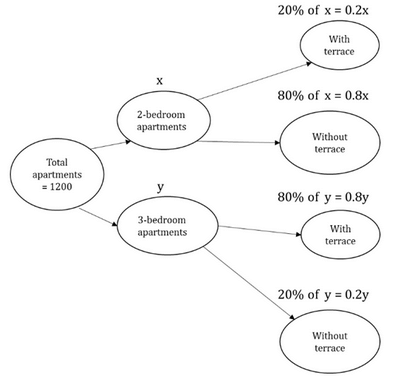Step 2: Finding required values

Now, we have two equations and two variables. So, let’s solve them to get the value of y.

x + y = 1200         ……………… (1)

2x + 8y = 6000      ……………… (2)

Multiplying equation (1) by 2, and then subtracting it from equation (2), we get:

6y = 3600

Thus,y = 600

Step 3: Calculating the final answer

So, the number of 3-bedroom apartments = 600

Test: Tree Structure - Question 7

In a day care, 80% of the kids are American.  60% of the kids have started speaking. Of these, only 20% are under the age of 2 years. If the number of non-American kids at the day care is 40, what is the number of kids who can speak and are not under 2 years of age?

Detailed Solution for Test: Tree Structure - Question 7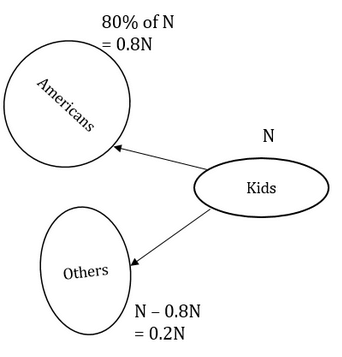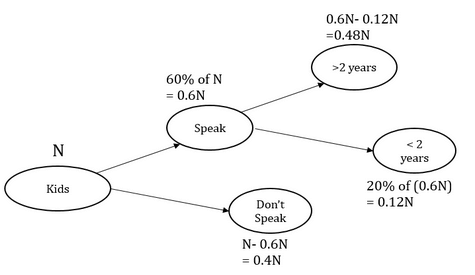Step 1: Question statement and Inferences

Let the total number of kids at the day care be N

So, number of American kids = 80% of N = 0.8N

Number of non-American kids = N-0.8N = 0.2N

It is given that 0.2N = 40

From here, we calculate that N = 200

Step 2: Finding required values

Number of kids who speak = 60% of N = 60% of 200 = 120

Of these, 20% are under 2 years of age.

Remaining 80% are not under 2 years of age

Step 3: Calculating the final answer

Number of kids who are not under 2 years of age = 80% of 120

= 96

Looking at the answer options, we see that option C is correct.

Test: Tree Structure - Question 8

If the students were awarded only three grades-A, B and C-how many students in the class got grade A?

(1) 20% of the class got grade B and 50% of the class got grade C.

(2) The 12 girls in the class make up 40% of the class.

a+b+c=T

Detailed Solution for Test: Tree Structure - Question 8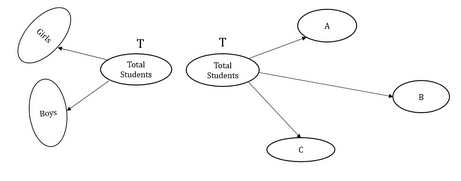Steps 1&2: Understand question and draw inferences

This question involves the creation of two trees.

In the first tree, the node ‘Total Students’ is branched on the basis of gender. So, we get two branches: Girls and Boys.

Let the number of Girls in the class be g, and the number of boys in the class be b.

Let the total number of students in the class be T.

So, we can write:

g+b=T

. . . Equation 1

In the second tree, the node ‘Total Students’ is branched out on the basis of grades.

Let the number of students who got grades A, B and C be a, b and c respectively.

So, we can write:

a+b+c=T. . . Equation 2

We need to find the value of a.

Step 3: Analyze Statement 1

As per Statement 1,

20% of the class got grade B and 50% of the class got grade C

b = 20% of T  and c = 50% of T

⇒ b = 0.2T and c = 0.5T

Substituting these values of b and c in Equation 2:

a + 0.2T + 0.5T = T

a=0.3 T

Since we don’t know the value of T, we will not be able to find the value of a.

Therefore, Statement 1 is not sufficient.

Step 4: Analyze Statement 2

As per Statement 2,

The 12 girls in the class make up 40% of the class

⇒ g=12

and g=40% of T

⇒  12 = 40100T

⇒ T = 30

However, this statement gives us no clue about the value of a. Therefore, this statement is clearly not sufficient.

Step 5: Analyze Statement 1 and Statement 2 together (if needed)

From Statement 1,

a=0.3 T

From Statement 2,

T = 30

Thus, by combining the two statements,

We get a = 9

Thus, we are able to get a unique value by combining the two statements.

Test: Tree Structure - Question 9

A premier Film and Performing Arts school has a total class strength of 770. Two-third of the male students and three-fourth of the female students have opted for acting courses. The remaining students have opted for other courses. If the number of female students who opted for other courses was 55 less, then there would be 2 male students who opted for other courses for every female student who opted for other courses. How many students have opted for the acting courses?

Detailed Solution for Test: Tree Structure - Question 9

Step 1: Question statement and Inferences

We are given that in a certain school, there are a total of 770 students. Let’s say the number of male students is x and the number of female students is y. So,

x + y = 770            …………… (1)

The number of male students in acting courses = 2x/3

The number of male students in other courses = x/3

The number of female students in acting courses = 3y/4

The number of female students in other courses = y/4

Thus,

The total number of students in acting courses =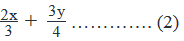And, the total number of students in other courses=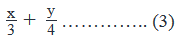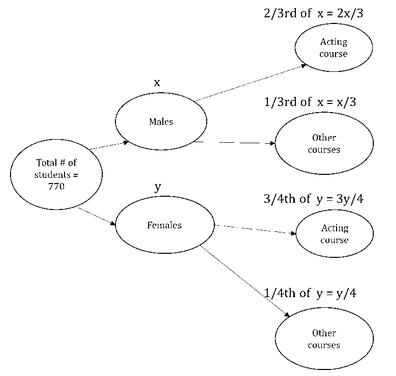It is also given that, If there were 55 less female students who opted for other courses, then the number of male students who opted for other courses will be twice that of the female students who opted for other courses. So,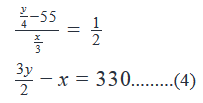Step 2: Finding required values

Adding equations (1) and (4), we get y = 440 from which we can calculate x = 330.

Step 3: Calculating the final answer

Now, from equation (2),

The total number of students in acting courses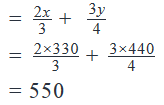Correct choice : D

Test: Tree Structure - Question 10

A certain university spends all the money donated by The Goodwill Foundation in a certain year to fund three research projects P, Q and R. Project P receives a funding of \$40,000. Both projects P and Q receive 100% more funding than Project R. The donation made by The Goodwill Foundation to the university is 25% of the total donation received by the university during the year. If the university invests in projects P, Q and R only from the money received from The Goodwill Foundation, then what is the total amount, in dollars, of donation that is not received by the university from The Goodwill Foundation?

Detailed Solution for Test: Tree Structure - Question 10

Step 1: Question statement and Inferences

The first step will be to draw the tree structure that depicts the question statement.

The next step will be to find the value at each node and branch of the tree.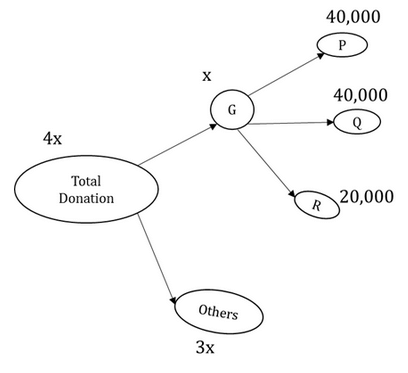Step 2: Finding required values

Let the money donated by The Goodwill Foundation be \$x

It is given that

Amount donated by Goodwill Foundation =25% of Total Donation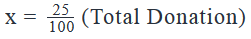Total Donation=4x

So, Donations by others=4x–x=3x

We need to find the value of

We are also given that

Also,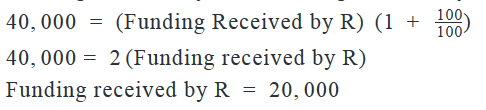Thus,x=40,000 + 40,000 + 20,000 = \$100,000

Step 3: Calculating the final answer

Donation that is not received by the university from The Goodwill Foundation = 3x = \$300,000

Test: Tree Structure - Question 11

Mid-way through their MBA, the students of the Class of 2012 in a business school were surveyed for career preferences. One-third of the students chose consulting as their first preference. When the survey was repeated upon completion of the MBA, it was found that two-third of the students who earlier preferred consulting had changed their mind. Among the students who chose consulting in both the surveys, three-fifths became entrepreneurs and opened consulting startups and the remaining 12 joined consulting firms as employees. How many students did the Class of 2012 have?

Detailed Solution for Test: Tree Structure - Question 11

Step 1: Question statement and Inferences

Let the number of students in the Class of 2012 be x.

So, number of students who chose consultancy in the first survey = x/3.

Two-third out of the students who chose consultancy as their first career choice, changed their mind by the completion of the course.

So, the number of students who changed their mind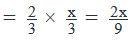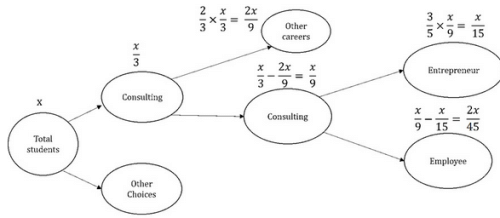Step 2: Finding required values

Out of the students who chose consulting in the second survey as well, three-fifth started their careers as entrepreneurs and 12 as employee to firms.

The number of students who still chose consulting in the second survey =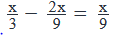The number of student who became entrepreneurs = 3/5 x The number of students who stayed with their first choice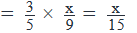The number of student who joined firms = 12

That is,Step 3: Calculating the final answer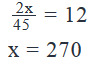Thus, the total number of students in the class = 270.

Test: Tree Structure - Question 12

In an interview for a certain job, 120 candidates appeared and two questions were asked to each candidate. How many candidates did not answer any question correctly?

(1) Of all the candidates who appeared for the interview, 20% of the candidates who answered at least one question correctly, answered both questions correctly.

(2) Of all the candidates who appeared for the interview, 25% of the candidates who answered none or both questions correctly, answered both questions correctly.

Detailed Solution for Test: Tree Structure - Question 12

Steps 1&2: Understand question and draw inferences

Let the number of candidates who gave 0 correct answers, 1 correct answer and 2 correct answers = A, B and C respectively

A + B + C = 120 -----(1)

We have to find the value of A.

To do so, we need to find:

(i)    Either the value of B and C

(ii)   Or the value of (B + C)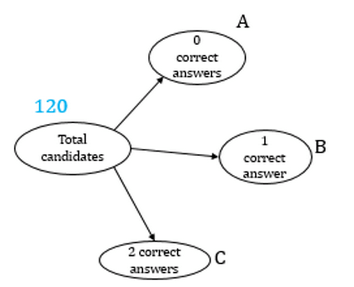Step 3: Analyze Statement 1

Number of students who answered at least one question correctly = B + C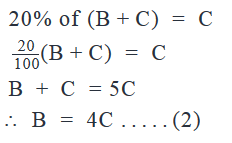We derive a relation between B and C, but we cannot find the value of B or C or (B + C) from equation (1) and (2).

INSUFFICIENT.

Step 4: Analyze Statement 2

Number of students who answered none or all questions correctly = A + C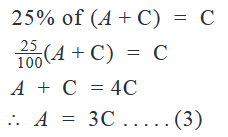We derive a relation between A and C, but we cannot find the value of B or C or (B + C) from equation (1) and (3).

Step 5: Analyze Statement 1 and Statement 2 together (if needed)

We have equations (1), (2) and (3) when we combine the two statements.

Substituting A and B from equation (2) and (3) in equation (1),

3C + 4C + C = 120

We can find the value of C from this equation and substitute the value in equation (3) the find the required value of A.

SUFFICIENT.

Test: Tree Structure - Question 13

If 80% employees of a company play soccer, what percent of the employees play golf?

(1) 25% of the employees who play soccer also play golf.

(2) 50% of the employees who play golf also play soccer.

Detailed Solution for Test: Tree Structure - Question 13

Steps 1 & 2: Understand Question and Draw Inferences

We are given that 80% employees of a company play soccer. We have to find the percentage of the employees who play golf.

Since this question only talks about the percentage, not the absolute numbers, let’s say the number of employees in the company is 100.

80% of these employees play soccer. Now, these 80% employees include the employees who play only soccer and the employees who play soccer and golf both.

So, the employees who play soccer = 80% of 100 = 80

Let’s say the number of employees who play both soccer and golf = x

Thus, the number of employees who play only soccer = 80 – x

Also, let the number of employees who play only golf = y

If there are any employees who play neither soccer nor golf = 20 – y

The total number of employees who play golf = x + y

We have to find the value of (x + y).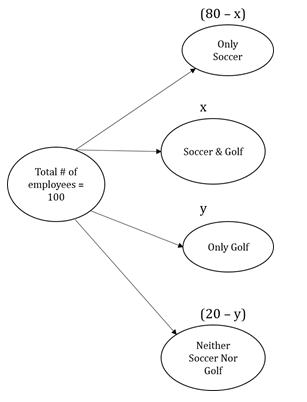Step 3: Analyze Statement 1

Statement (1) says: 25% of the employees who play soccer also play golf.

The number of employees who play soccer and golf is x.

Per this statement:

x = 25% of 80

x = 20   ………….. (1)

However, since we do not know the value of y, we cannot get the value of (x + y).

Hence, statement (1) is not sufficient to answer the question: What is the value of (x + y)?

Step 4: Analyze Statement 2

Statement (2) says:  50% of the employees who play golf also play soccer.

The employees who play golf and soccer are (x + y). 50% of these employees also play soccer. Out of these (x + y) employees, the employees who play soccer = x

50% of (x + y) = x

x + y = 2x

y = x             …… (2)

However, we don’t know the value of x and y. so, we can’t determine the value of (x + y).

Hence, statement II is not sufficient to answer the question: What is the value of (x + y)?

Step 5: Analyze Both Statements Together (if needed)

Since step 3 and step 4 were not able to provide the answer to the question, let’s analyse both the statements together:

Statement (1):

x = 20   ………….. (1)

Statement (2):

y = x          ………… (2)

Thus,

y = 20

Hence,

x + y = 20 + 20 = 40

So, statement (1) and (2) combined are sufficient to answer the question.

## Integrated Reasoning for GMAT

24 videos|33 docs|24 tests
 Use Code STAYHOME200 and get INR 200 additional OFF Use Coupon Code
Information about Test: Tree Structure Page
In this test you can find the Exam questions for Test: Tree Structure solved & explained in the simplest way possible. Besides giving Questions and answers for Test: Tree Structure, EduRev gives you an ample number of Online tests for practice

## Integrated Reasoning for GMAT

24 videos|33 docs|24 tests